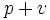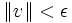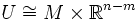# Tubular neighborhood theorem

Jump to: navigation, search
This fact is an application of the following pivotal fact/result/idea: inverse function theorem
View other applications of inverse function theorem OR Read a survey article on applying inverse function theorem
This fact is an application of the following pivotal fact/result/idea: existence of smooth partitions of unity
View other applications of existence of smooth partitions of unity OR Read a survey article on applying existence of smooth partitions of unity
This fact is an application of the following pivotal fact/result/idea: Lebesgue number lemma
View other applications of Lebesgue number lemma OR Read a survey article on applying Lebesgue number lemma

## Statement

Let$M$ be a submanifold (differential sense) of$\R^n$, of dimension$m$. Then, there exists$\epsilon>0$ such that for any point at distance at most$\epsilon$ from$M$, there is a unique expression of the point as a sum$p + v$ where$p \in M$ and$v$ is a normal at$p$, with$\| v \| < \epsilon$.

If we define$U$ as the open subset of$\R^n$ comprising those points of$\R^n$ at distance less than$\epsilon$ from$M$, then$U$ can be viewed as a concrete realization, in the ambient space$\R^n$, of the normal bundle to$M$ in$\R^n$. In the situations where the normal bundle to$M$ is trivial, we see that this gives a natural diffeomorphism$U \cong M \times \R^{n-m}$.